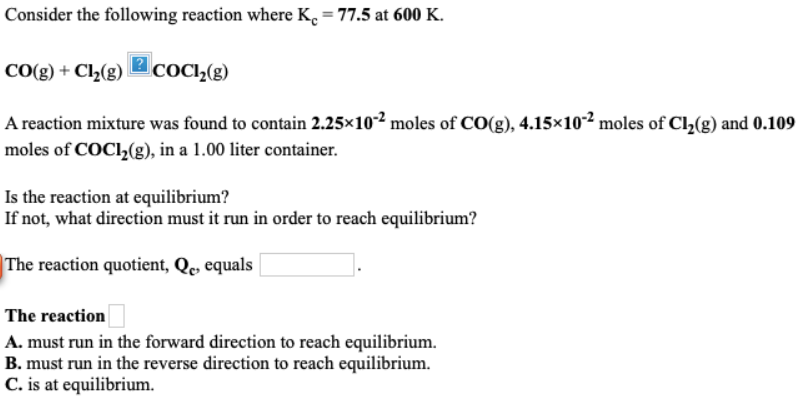# Consider the following reaction where Kc = 77.5 at 600 K. CO(g) + Cl2(g) -> COCl2(g) A reaction mixture was found to contain 2.25 x 10^-2 moles of CO(g), 4.15 x 10^-2 moles of Cl2(g), and 0.109 moles of COCl2(g), in a 1.00 liter container. Is the reaction at equilibrium? If not, what direction must it run in order to reach equilibrium? The reaction quotient, Qc, equals ___. The reaction a. must run in the forward direction to reach equilibrium. b. must run in the reverse direction to reach equilibrium. c. is at equilibrium.